# Greybox main vignette

#### 2022-03-24

There are three well-known notions of “boxes” in modelling: 1. White box - the model that is completely transparent and does not have any randomness. One can see how the inputs are transformed into the specific outputs. 2. Black box - the model which does not have an apparent structure. One can only observe inputs and outputs but does not know what happens inside. 3. Grey box - the model that is in between the first two. We observe inputs and outputs plus have some information about the structure of the model, but there is still a part of unknown.

The white boxes are usually used in optimisations (e.g. linear programming), while black boxes are popular in machine learning. As for the grey box models, they are more often used in analysis and forecasting. So the package greybox contains models that are used for these purposes.

At the moment the package contains augmented linear model function and several basic functions that implement model selection and combinations using information criteria (IC). You won’t find statistical tests in this package - there’s plenty of them in the other packages. Here we try using the modern techniques and methods that do not rely on hypothesis testing. This is the main philosophical point of greybox.

## Main functions

The package includes the following functions for models construction:

1. alm() - Augmented Linear Model. This is something similar to GLM, but with a focus on forecasting and the information criteria usage for time series. It also supports mixture distribution models for the intermittent data and allows adding trend to the data via the formula.
2. stepwise() - select the linear model with the lowest IC from all the possible in the provided data. Uses partial correlations. Works fast;
3. lmCombine() - combine the linear models into one using IC weights;
4. lmDynamic() - produce model with dynamic weights and time varying parameters based on point IC weight.

See discussion of some of these functions in this vignette below.

## Models evaluation functions

1. ro() - produce forecasts with a specified function using rolling origin.
2. measures() - function, returning a bunch of error measures for the provided forecast and the holdout sample.
3. rmcb() - regression on ranks of forecasting methods. This is a fast alternative to the classical nemenyi / MCB test.

## Marketing analytics tools

1. tableplot() - creates a plot for two categorical variables based on table function with frequencies inside.
2. cramer() - Cramer’s V value.
3. mcor() - the multiple correlation coefficient.
4. association() - the matrix of measures of association.
5. spread() - function that plots scatter / boxplot / tableplot diagrams between variables depending on the their types.
6. determination() - coefficients of determination for the set of explanatory variables.

All these functions are discussed in a separate vignette on marketing analytics tools.

## Methods

The following methods can be applied to the models, produced by alm(), stepwise(), lmCombine() and lmDynamic():

1. logLik() - extracts log-likelihood.
2. AIC(), AICc(), BIC(), BICc() - calculates the respective information criteria.
3. pointLik() - extracts the point likelihood.
4. pAIC(), pAICc(), pBIC(), pBICc() - calculates the respective point information criteria, based on pointLik.
5. actuals() - extracts the actual values of the response variable.
6. coefbootstrap() - produces bootstrapped values of parameters, taking nsim samples of the size size from the data and reapplying the model.
7. coef(), coefficients() - extract the parameters of the model.
8. confint() - extracts the confidence intervals for the parameters.
9. vcov() - extracts the variance-covariance matrix of the parameters.
10. sigma() - extracts the standard deviation of the residuals.
11. nobs() - the number of the in-sample observations of the model.
12. nparam() - the number of all the estimated parameters in the model.
13. nvariate() - the number of variates (columns / dimensions) of the resposne variable.
14. summary() - produces the summary of the model.
15. predict() - produces the predictions based on the model and the provided newdata. If the newdata is not provided, then it uses the already available data in the model. Can also produce confidence and prediction intervals.
16. forecast() - acts similarly to predict() with few differences. It has a parameter h - forecast horizon - which is NULL by default and is set to be equal to the number of rows in newdata. However, if the newdata is not provided, then it will produce forecasts of the explanatory variables to the horizon h and use them as newdata. Finally, if h and newdata are provided, then the number of rows to use will be regulated by h.
17. plot() - produces several plots for the analysis of the residuals. This includes: Fitted over time, Standardised residuals vs Fitted, Absolute residuals vs Fitted, Q-Q plot with the specified distribution, Squared residuals vs Fitted, ACF of the residuals and PACF of the residuals, which is regulated by which parameter. See documentation for more info: ?plot.greybox.
18. detectdst() and detectleap() - methods that return the ids of the hour / date for the DST / Leap year change.
19. extract() method, needed in order to produce printable regression outputs using texreg() function from the texreg package.

## Exogenous variables transformation tools

1. xregExpander() - expand the provided data by including leads and lags of the variables. See details below.
2. xregTransformer() - produce non-linear transformations of the provided data (logs, inverse etc).
3. xregMultiplier() - produce cross-products of the variables in the provided matrix. Could be useful when exploring interaction effects of dummy variables.
4. temporaldummy() - the method that generates a matrix with dummy variables based on the provided object and selected type and of. Can be handy, when you want to construct a regression with dummies for a time series object (e.g. zoo).
5. outlierdummy() - the method that creates a matrix of dummy variables based on the residuals of an object, selected confidence level and type of residuals.

## Distribution functions

1. qlaplace(), dlaplace(), rlaplace(), plaplace() - functions for Laplace distribution.
2. qalaplace(), dalaplace(), ralaplace(), palaplace() - functions for Asymmetric Laplace distribution.
3. qs(), ds(), rs(), ps() - functions for S distribution.
4. qgnorm(), dgnorm(), rgnorm(), pgnorm() - functions for the Generalised normal distribution.
5. qfnorm(), dfnorm(), rfnorm(), pfnorm() - functions for folded normal distribution.
6. qtplnorm(), dtplnorm(), rtplnorm(), ptplnorm() - functions for three parameter log normal distribution.
7. qbcnorm(), dbcnorm(), rbcnorm(), pbcnorm() - functions for the Box-Cox normal distribution.
8. qlogitnorm(), dlogitnorm(), rlogitnorm(), plogitnorm() - functions for the Logit-normal distribution.

1. graphmaker() - produces linear plots for the variable, its forecasts and fitted values.

## xregExpander

The function xregExpander() is useful in cases when the exogenous variable may influence the response variable either via some lags or leads. As an example, consider BJsales.lead series from the datasets package. Let’s assume that the BJsales variable is driven by the today’s value of the indicator, the value five and 10 days ago. This means that we need to produce lags of BJsales.lead. This can be done using xregExpander():

BJxreg <- xregExpander(BJsales.lead,lags=c(-5,-10))

The BJxreg is a matrix, which contains the original data, the data with the lag 5 and the data with the lag 10. However, if we just move the original data several observations ahead or backwards, we will have missing values in the beginning / end of series, so xregExpander() fills in those values with the forecasts using es() and iss() functions from smooth package (depending on the type of variable we are dealing with). This also means that in cases of binary variables you may have weird averaged values as forecasts (e.g. 0.7812), so beware and look at the produced matrix. Maybe in your case it makes sense to just substitute these weird numbers with zeroes…

You may also need leads instead of lags. This is regulated with the same lags parameter but with positive values:

BJxreg <- xregExpander(BJsales.lead,lags=c(7,-5,-10))

Once again, the values are shifted, and now the first 7 values are backcasted. In order to simplify things we can produce all the values from 10 lags till 10 leads, which returns the matrix with 21 variables:

BJxreg <- xregExpander(BJsales.lead,lags=c(-10:10))

## stepwise

The function stepwise() does the selection based on an information criterion (specified by user) and partial correlations. In order to run this function the response variable needs to be in the first column of the provided matrix. The idea of the function is simple, it works iteratively the following way:

1. The basic model of the first variable and the constant is constructed (this corresponds to simple mean). An information criterion is calculated;
2. The correlations of the residuals of the model with all the original exogenous variables are calculated;
3. The regression model of the response variable and all the variables in the previous model plus the new most correlated variable from (2) is constructed using lm() function;
4. An information criterion is calculated and is compared with the one from the previous model. If it is greater or equal to the previous one, then we stop and use the previous model. Otherwise we go to step 2.

This way we do not do a blind search, going forward or backwards, but we follow some sort of “trace” of a good model: if the residuals contain a significant part of variance that can be explained by one of the exogenous variables, then that variable is included in the model. Following partial correlations makes sure that we include only meaningful (from technical point of view) variables in the model. In general the function guarantees that you will have the model with the lowest information criterion. However this does not guarantee that you will end up with a meaningful model or with a model that produces the most accurate forecasts. So analyse what you get as a result.

Let’s see how the function works with the Box-Jenkins data. First we expand the data and form the matrix with all the variables:

BJxreg <- as.data.frame(xregExpander(BJsales.lead,lags=c(-10:10)))
BJxreg <- cbind(as.matrix(BJsales),BJxreg)
colnames(BJxreg) <- "y"
ourModel <- stepwise(BJxreg)

This way we have a nice data frame with nice names, not something weird with strange long names. It is important to note that the response variable should be in the first column of the resulting matrix. After that we use stepwise function:

ourModel <- stepwise(BJxreg)

And here’s what it returns (the object of class lm):

ourModel
#> Time elapsed: 0.07 seconds
#> Call:
#> alm(formula = y ~ xLag4 + xLag9 + xLag3 + xLag10 + xLag5 + xLag6 +
#>     xLead9 + xLag7 + xLag8, data = data, distribution = "dnorm")
#>
#> Coefficients:
#> (Intercept)       xLag4       xLag9       xLag3      xLag10       xLag5
#>  17.6668913   3.3703773   1.3725674   4.6768101   1.5415708   2.3209845
#>       xLag6      xLead9       xLag7       xLag8
#>   1.7074084   0.3765307   1.4027852   1.3371727

The values in the function are listed in the order of most correlated with the response variable to the least correlated ones. The function works very fast because it does not need to go through all the variables and their combinations in the dataset.

All the basic methods can be used together with the final model (e.g. predict(), forecast(), summary() etc).

Furthermore, the greybox package implements extract() method from texreg package for the production of printable outputs from the regression, here is an example:

texreg::htmlreg(ourModel)
Statistical models
Model 1
(Intercept) 17.67*
[16.07; 19.26]
xLag4 3.37*
[ 2.75; 3.99]
xLag9 1.37*
[ 0.75; 1.99]
xLag3 4.68*
[ 4.10; 5.25]
xLag10 1.54*
[ 0.98; 2.11]
xLag5 2.32*
[ 1.68; 2.96]
xLag6 1.71*
[ 1.06; 2.35]
[ 0.12; 0.63]
xLag7 1.40*
[ 0.76; 2.05]
xLag8 1.34*
[ 0.69; 1.98]
Num. obs. 150.00
Num. param. 11.00
Num. df 139.00
AIC 416.45
AICc 418.36
BIC 449.57
BICc 454.36
* 0 outside the confidence interval.

Similarly, you can produce pdf tables via texreg() function from that package. Alternatively, you can use kable() function from knitr package on the summary to get a table for LaTeX / HTML.

## lmCombine

lmCombine() function creates a pool of linear models using lm(), writes down the parameters, standard errors and information criteria and then combines the models using IC weights. The resulting model is of the class “lm.combined.” The speed of the function deteriorates exponentially with the increase of the number of variables $$k$$ in the dataset, because the number of combined models is equal to $$2^k$$. The advanced mechanism that uses stepwise() and removes a large chunk of redundant models is also implemented in the function and can be switched using bruteforce parameter.

Here’s an example of the reduced data with combined model and the parameter bruteforce=TRUE:

ourModel <- lmCombine(BJxreg[,-c(3:7,18:22)],bruteforce=TRUE)
summary(ourModel)
#> The AICc combined model
#> Response variable: y
#> Distribution used in the estimation: Normal
#> Coefficients:
#>             Estimate Std. Error Importance Lower 2.5% Upper 97.5%
#> (Intercept)  20.9213     0.2326     1.0000    20.4616     21.3811 *
#> x            -0.0432     0.0286     0.2591    -0.0998      0.0134
#> xLag5         6.3977     0.0839     1.0000     6.2318      6.5636 *
#> xLag4         5.8466     0.0899     1.0000     5.6688      6.0244 *
#> xLag3         5.6854     0.0901     1.0000     5.5074      5.8635 *
#> xLag2         0.1251     0.0382     0.2876     0.0496      0.2006 *
#> xLag1        -0.0844     0.0342     0.2717    -0.1521     -0.0167 *
#> xLead1       -0.0908     0.0324     0.2782    -0.1547     -0.0268 *
#> xLead2       -0.0356     0.0257     0.2600    -0.0865      0.0152
#> xLead3       -0.1200     0.0343     0.2971    -0.1877     -0.0523 *
#> xLead4       -0.0068     0.0229     0.2585    -0.0520      0.0384
#> xLead5        0.1159     0.0300     0.3030     0.0566      0.1751 *
#>
#> Error standard deviation: 2.2075
#> Sample size: 150
#> Number of estimated parameters: 7.2151
#> Number of degrees of freedom: 142.7849
#> Approximate combined information criteria:
#>      AIC     AICc      BIC     BICc
#> 670.6603 671.4964 692.3824 694.4770

summary() function provides the table with the parameters, their standard errors, their relative importance and the 95% confidence intervals. Relative importance indicates in how many cases the variable was included in the model with high weight. So, in the example above variables xLag5, xLag4, xLag3 were included in the models with the highest weights, while all the others were in the models with lower ones. This may indicate that only these variables are needed for the purposes of analysis and forecasting.

The more realistic situation is when the number of variables is high. In the following example we use the data with 21 variables. So if we use brute force and estimate every model in the dataset, we will end up with $$2^{21}$$ = 2^21 combinations of models, which is not possible to estimate in the adequate time. That is why we use bruteforce=FALSE:

ourModel <- lmCombine(BJxreg,bruteforce=FALSE)
summary(ourModel)
#> The AICc combined model
#> Response variable: y
#> Distribution used in the estimation: Normal
#> Coefficients:
#>             Estimate Std. Error Importance Lower 2.5% Upper 97.5%
#> (Intercept)  17.6924     0.7760     1.0000    16.1580     19.2268 *
#> xLag4         3.3747     0.3020     1.0000     2.7775      3.9719 *
#> xLag9         1.3711     0.3029     0.9998     0.7723      1.9699 *
#> xLag3         4.6846     0.2809     1.0000     4.1292      5.2400 *
#> xLag10        1.5424     0.2749     1.0000     0.9989      2.0859 *
#> xLag5         2.3222     0.3118     1.0000     1.7058      2.9386 *
#> xLag6         1.7075     0.3145     1.0000     1.0858      2.3293 *
#> xLead9        0.3638     0.1247     0.9663     0.1174      0.6103 *
#> xLag7         1.4017     0.3152     0.9997     0.7786      2.0249 *
#> xLag8         1.3363     0.3132     0.9994     0.7170      1.9556 *
#>
#> Error standard deviation: 0.936
#> Sample size: 150
#> Number of estimated parameters: 10.9652
#> Number of degrees of freedom: 139.0348
#> Approximate combined information criteria:
#>      AIC     AICc      BIC     BICc
#> 416.7030 418.6040 449.7152 454.4777

In this case first, the stepwise() function is used, which finds the best model in the pool. Then each variable that is not in the model is added to the model and then removed iteratively. IC, parameters values and standard errors are all written down for each of these expanded models. Finally, in a similar manner each variable is removed from the optimal model and then added back. As a result the pool of combined models becomes much smaller than it could be in case of the brute force, but it contains only meaningful models, that are close to the optimal. The rationale for this is that the marginal contribution of variables deteriorates with the increase of the number of parameters in case of the stepwise function, and the IC weights become close to each other around the optimal model. So, whenever the models are combined, there is a lot of redundant models with very low weights. By using the mechanism described above we remove those redundant models.

There are several methods for the lm.combined class, including:

1. predict.greybox() - returns the point and interval predictions.
2. forecast.greybox() - wrapper around predict() The forecast horizon is defined by the length of the provided sample of newdata.
3. plot.lm.combined() - plots actuals and fitted values.
4. plot.predict.greybox() - which uses graphmaker() function from smooth in order to produce graphs of actuals and forecasts.

As an example, let’s split the whole sample with Box-Jenkins data into in-sample and the holdout:

BJInsample <- BJxreg[1:130,];
BJHoldout <- BJxreg[-(1:130),];
ourModel <- lmCombine(BJInsample,bruteforce=FALSE)

A summary and a plot of the model:

summary(ourModel)
#> The AICc combined model
#> Response variable: y
#> Distribution used in the estimation: Normal
#> Coefficients:
#>             Estimate Std. Error Importance Lower 2.5% Upper 97.5%
#> (Intercept)  19.4134     0.8558     1.0000    17.7189     21.1079 *
#> xLag4         3.3480     0.2966     1.0000     2.7607      3.9353 *
#> xLag9         1.3340     0.2983     0.9990     0.7434      1.9247 *
#> xLag3         4.7559     0.2787     1.0000     4.2040      5.3078 *
#> xLag10        1.5364     0.2701     1.0000     1.0016      2.0713 *
#> xLag5         2.3209     0.3063     1.0000     1.7144      2.9274 *
#> xLag6         1.6611     0.3090     1.0000     1.0493      2.2730 *
#> xLead9        0.2948     0.1260     0.8920     0.0454      0.5443 *
#> xLag8         1.3692     0.3084     0.9989     0.7585      1.9799 *
#> xLag7         1.3274     0.3093     0.9982     0.7150      1.9397 *
#>
#> Error standard deviation: 0.9541
#> Sample size: 130
#> Number of estimated parameters: 10.8881
#> Number of degrees of freedom: 119.1119
#> Approximate combined information criteria:
#>      AIC     AICc      BIC     BICc
#> 367.7981 369.9900 399.0203 404.3546
plot(ourModel)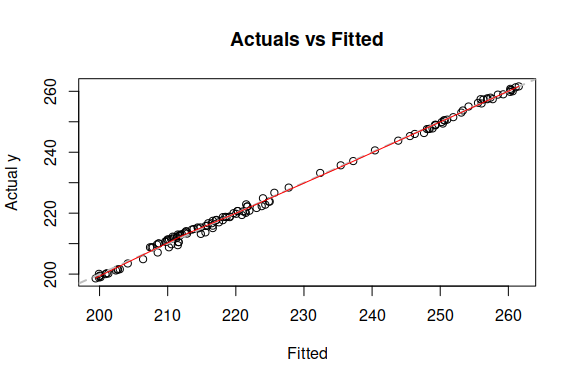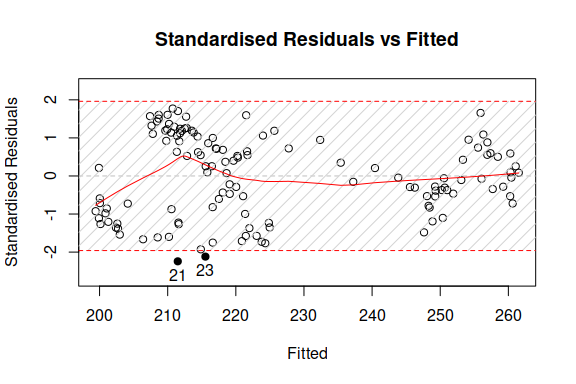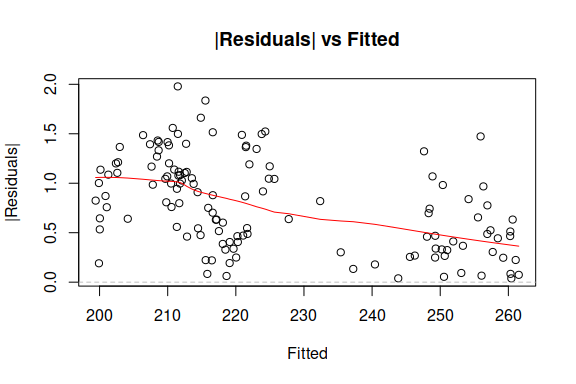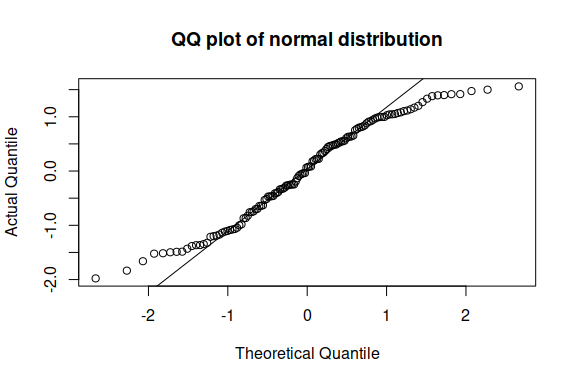Importance tells us how important the respective variable is in the combination. 1 means 100% important, 0 means not important at all.

And the forecast using the holdout sample:

ourForecast <- predict(ourModel,BJHoldout)
plot(ourForecast)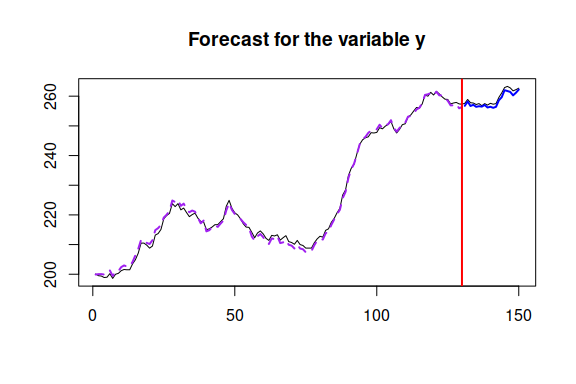These are the main functions implemented in the package for now. If you want to read more about IC model selection and combinations, I would recommend (Burnham and Anderson 2004) textbook.